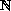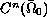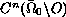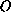Electron. J. Diff. Eqns., Vol. 2001(2001), No. 01, pp. 1-26.

### On the singularities of 3-D Protter's problem for the wave equation Myron K. Grammatikopoulos, Tzvetan D. Hristov, & Nedyu I. Popivanov

Abstract:
In this paper we study boundary-value problems for the wave equation, which are three-dimensional analogue of Darboux-problems (or of Cauchy-Goursat problems) on the plane. It is shown that forinthere exists a right hand side smooth function from, for which the corresponding unique generalized solution belongs to, and it has a strong power-type singularity at the point. This singularity is isolated at the vertexof the characteristic cone and does not propagate along the cone. In this paper we investigate the behavior of the singular solutions at the point. Also, we study more general boundary-value problems and find that there exist an infinite number of smooth right-hand side functions for which the corresponding unique generalized solutions are singular. Some a priori estimates are also stated.

Submitted October 30, 2000. Published January 1, 2001.
Math Subject Classifications: 35L05, 35L20, 35D05, 35A20.
Key Words: Wave equation, boundary-value problems, generalized solution, singular solutions, propagation of singularities.

Show me the PDF file (243K), TEX file, and other files for this article.Myron K. Grammatikopoulos Department of Mathematics University of Ioannina 451 10 Ioannina, Greece email: mgrammat@cc.uoi.gr Tzvetan D. Hristov Institute of Mathematics and Informatics Bulgarian Academy of Sciences 1113 Sofia, Bulgaria email: tzvetan@math.bas.bg Nedyu I. Popivanov Department of Mathematics and Informatics University of Sofia 1164 Sofia, Bulgaria email: nedyu@fmi.uni-sofia.bg